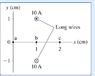# Magnetic Field at Points A, B & C in Figure

• aloko
In summary, the magnitude of the magnetic field at point a is 0 T, the direction is to the right. The magnitude of the magnetic field at point b is 2*10^-6 T, with the direction to the left. Finally, the magnitude of the magnetic field at point c is 1*10^-6 T, with the direction upwards.

#### aloko

a. What is the magnitude of the magnetic field at point a in the figure?

b. What is the direction of the magnetic field at point a in the figure?

c. What is the magnitude of the magnetic field at point b in the figure?

d. What is the direction of the magnetic field at point b in the figure?

e. What is the magnitude of the magnetic field at point c in the figure?

c. What is the direction of the magnetic field at point c in the figure?

Thanks

#### Attachments

•magnetic field question.PNG
3.5 KB · Views: 359
Hi aloko, welcome to PF.
Before you ask our help, you have search for the relevant equations. Show your attempts. If you don't get the answer, we will see where you have made mistake.
So proceed.

Attempted solution

a. for magnetic field B at point a,

magnetic field, B=U*I/[2*pie*r], where U=4*pie*10^-7, r is the radius, I=current

so, magnetic field at point a is; B= [(4*3.14*10^-7)*10]/[(2*3.14*0)]
therefore B=0 T

b. direction of the mag. field is to the right.

c. magnetic field B at point b,

B= [(4*3.14*10^-7)*10]/[2*3.14*1]= 2*10^-6 T

d. direction of the mag. field is to the left

e. magnetic field, B at point C

B= [(4*3.14*10^-7)*10]/[2*3.14*2]
B=1*10^-6 T

f. direction of the mag. field is up.